# Excel IF statement between two numbers or dates

The tutorial shows how to use an Excel IF formula to see if a given number or date falls between two values.

To check if a given value is between two numeric values, you can use the AND function with two logical tests. To return your own values when both expressions evaluate to TRUE, nest AND inside the IF function. Detailed examples follow below.

## Excel formula: if between two numbers

To test if a given number is between two numbers that you specify, use the AND function with two logical tests:

• Use the greater then (>) operator to check if the value is higher than a smaller number.
• Use the less than (<) operator to check if the value is lower than a larger number.

The generic If between formula is:

AND(value > smaller_number, value < larger_number)

To include the boundary values, use the greater than or equal to (>=) and less than or equal to (<=) operators:

AND(value >= smaller_number, value <= larger_number)

For example, to see if a number in A2 falls between 10 and 20, not including the boundary values, the formula in B2, copied down, is:

`=AND(A2>10, A2<20)`

To check if A2 is between 10 and 20, including the threshold values, the formula in C2 takes this form:

`=AND(A2>=10, A2<=20)`

In both cases, the result is the Boolean value TRUE if the tested number is between 10 and 20, FALSE if it is not: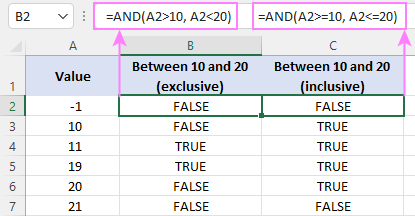### If between two numbers then

In case you want to return a custom value if a number is between two values, then place the AND formula in the logical test of the IF function.

For example, to return "Yes" if the number in A2 is between 10 and 20, "No" otherwise, use one of these IF statements:

If between 10 and 20:

`=IF(AND(A2>10, A2<20), "Yes", "No")`

If between 10 and 20, including the boundaries:

`=IF(AND(A2>=10, A2<=20), "Yes", "No")`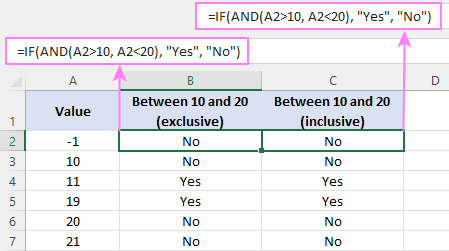Tip. Instead of hardcoding the threshold values in the formula, you can input them in individual cells, and refer to those cells like shown in the below example.

Suppose you have a set of values in column A and wish to know which of the values fall between the numbers in columns B and C in the same row. Assuming a smaller number is always in column B and a larger number is in column C, the task can be accomplished with this formula:

`=IF(AND(A2>B2, A2<C2), "Yes", "No")`

Including the boundaries:

`=IF(AND(A2>=B2, A2<=C2), "Yes", "No")`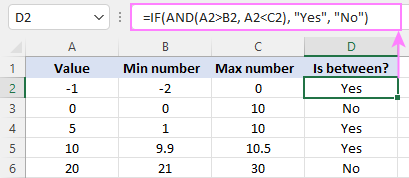And here is a variation of the If between statement that returns a value itself if TRUE, some text or an empty string if FALSE:

`=IF(AND(A2>10, A2<20), A2, "Invalid")`

Including the boundaries:

`=IF(AND(A2>=10, A2<=20), A2, "Invalid")`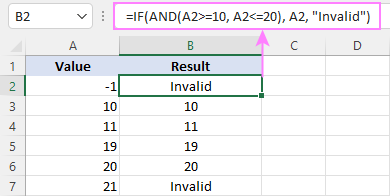### If boundary values are in different columns

When smaller and larger numbers you are comparing against may appear in different columns (i.e. number 1 is not always smaller than number 2), use a slightly more complex version of the formula.

AND(value > MIN(num1, num2), value < MAX(num1, num2))

Here, we first test if the target value is higher than a smaller of the two numbers returned by the MIN function, and then check if it is lower than a larger of the two numbers returned by the MAX function.

To include the threshold numbers, adjust the logic as follows:

AND(value >= MIN(num1, num2), value <= MAX(num1, num2))

For example, to find out if a number in A2 falls between two numbers in B2 and C2, use one of these formulas:

Excluding boundaries:

`=AND(A2>MIN(B2, C2), A2<MAX(B2, C2))`

Including boundaries:

`=AND(A2>=MIN(B2, C2), A2<=MAX(B2, C2))`

To return your own values instead of TRUE and FALSE, use the following Excel IF statement between two numbers:

`=IF(AND(A2>MIN(B2, C2), A2<MAX(B2, C2)), "Yes", "No")`

Or

`=IF(AND(A2>=MIN(B2, C2), A2<=MAX(B2, C2)), "Yes", "No")`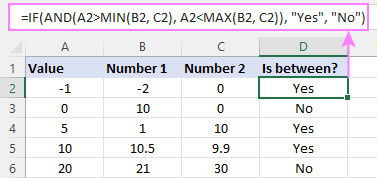## Excel formula: if between two dates

The If between dates formula in Excel is essentially the same as If between numbers.

To check whether a given date is within a certain range, the generic formula is:

IF(AND(date >= start_date, date <= end_date), value_if_true, value_if_false)

Not including the boundary dates:

IF(AND(date > start_date, date < end_date), value_if_true, value_if_false)

However, there is a caveat: IF does recognize dates supplied directly to its arguments and regards them as text strings. For IF to recognize a date, it should be wrapped in the DATEVALUE function.

For example, to test if a date in A2 falls between 1-Jan-2022 and 31-Dec-2022 inclusive, you can use this formula:

`=IF(AND(A2>=DATEVALUE("1/1/2022"), A2<=DATEVALUE("12/31/2022")), "Yes", "No")`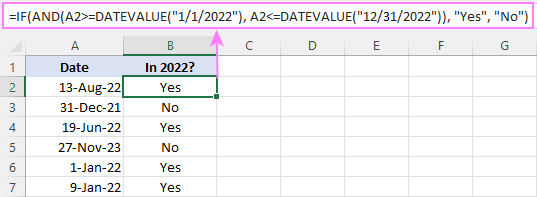In case, the start and end dates are in predefined cells, the formula becomes much simpler:

`=IF(AND(A2>=\$E\$2, A2<=\$E\$3), "Yes", "No")`

Where \$E\$2 is the start date and \$E\$3 is the end date. Please notice the use of absolute references to lock the cell addresses, so the formula won't break when copied to the below cells.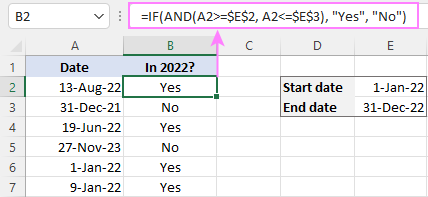Tip. If each tested date should fall in its own range, and the boundary dates may be interchanged, then use the MIN and MAX functions to determine a smaller and larger date as explained in If boundary values are in different columns.

### If date is within next N days

To test if a date is within the next n days of today's date, use the TODAY function to determine the start and end dates. Inside the AND statement, the first logical test checks if the target date is greater than today's date, while the second logical test checks if it is less than or equal to the current date plus n days:

IF(AND(date > TODAY(), date <= TODAY()+n), value_if_true, value_if_false)

For example, to test if a date in A2 occurs in the next 7 days, the formula is:

`=IF(AND(A2>TODAY(), A2<=TODAY()+7), "Yes", "No")`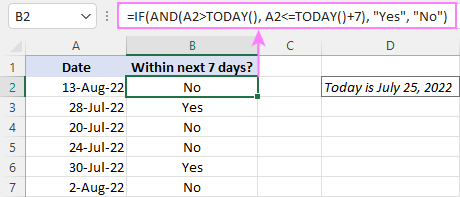### If date is within last N days

To test if a given date is within the last n days of today's date, you again use IF together with the AND and TODAY functions. The first logical test of AND checks if a tested date is greater than or equal to today's date minus n days, and the second logical test checks if the date is less than today:

IF(AND(date >= TODAY()-n, date < TODAY()), value_if_true, value_if_false)

For example, to determine if a date in A2 occurred in the last 7 days, the formula is:

`=IF(AND(A2>=TODAY()-7, A2<TODAY()), "Yes", "No")`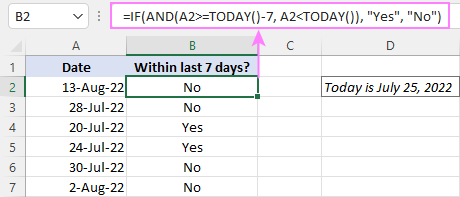Hopefully, our examples have helped you understand how to use the If between formula in Excel efficiently. I thank you for reading and hope to see you on our blog next week!

## Practice workbook

Excel If between - formula examples (.xlsx file)

## You may also be interested in

1. Hi Alex,
I am trying to determine if the current time (cell D3: =now() - formulated to XX:XX AM/PM) is between a shift start and a shift end time and return either "on-shift" or "off-shift". I am using these two formulas:
1/ =IF(AND(\$D\$3>D31, \$D\$3MIN(D23,E23),\$D\$3<MAX(D23,E23)), "On-shift","Off-shift") -- only gives "Off shift even when current time is between shift start and end time.

• Hi! I don't know what data is written in cells D31, D23, E23. Therefore, I cannot check your formula.

2. I am getting "false" message for below formula. This is used to find out two days differance to find total number of days

IF((\$P\$3-\$O7)>=60,IF((\$P\$3-\$O7)<90,\$F7,0))

• FALSE means that none of your conditions in the IF formula are met. Check your data.

3. Trying to figure out how to produce this (not working)sheet has 2 tabs =IF column H matches column E (Names Match) on other tab (tab name) then check column D (Renewal Date) if less than or greater than date in column I5 (Jan-24) than produce column N Price or column O price

4. Hi,

I'm really struggling to find the way to do this, have tried multiple options in your guidance - but basically, I have two date columns and I want a series of different comments back - not simly late/on time - but four different options.

Column 1 is the due date; column 2 is the completed date, where a date only appears when the task is completed. I want to return:

if the completed date column is blank, but the due date has passed - return "open and late"
If the completed date is blank, but the due date not yet passed - return "open and on time"
If the completed date is entered but is later than the due date - return "closed late"
If the completed date is earlier than the due date - return "Closed on time"

So the two values option won't work IF(N1>K1, "completed late","on time") as it doesn't cover all the options and I tried nested variations but it seemed to think if the completed date was blank, it was on time, even when the due date was passed. It's the blank that I'm struggling - if it's blank, it depends on whether the due date is still live or has passsed as to what's returned. Many thanks

• Apols - also the IF(X>Y, "completed late","on time") also doesn't always work with the blanks. So where the completed column is blank, often it brings back on time, even when the due date has actually passed.

• Hi! Try to use the recommendations described in this article: Excel Nested IF statement: examples, best practices and alternatives. Check the empty cell using the ISBLANK function. If I got you right, the formula below will help you with your task:

=IF(AND(ISBLANK(B1),TODAY()>A1),"open and late", IF(AND(ISBLANK(B1),TODAY()<A1),"open and on time", IF(AND(NOT(ISBLANK(B1)),B1>A1),"closed late", IF(AND(NOT(ISBLANK(B1)),B1<A1),"closed on time",""))))

Hope this is what you need.

5. Hi Alex ,
thank you very much for your time , can you please help me with my formula please , all what I need to show is meet, not meet & (Blank if there is no date i the K columns) I did the Meet & not Meet formula but I just can't get the cell to be blank if there is no date entered , can you help me please , this is the formula I'm using =IF(K5>H5,"Meet",IF(K5<=I5,"Not Meet", I tried the IFBLANK ,"" , but nothing is working , what I need , when I enter date in K, column L will shoe me If meet and didn't meet (WORDs) the due date in (I) , but if there is no date in K I want L to be Blank,, can you help me please ..Thank you

H I J K L
Dyployment Date Lodgement due date Reminings days from today loedgment date Deadline were meet?
1/09/2023 6/09/2023 13 4/09/2023 Meet

• Hi! If I understand your task correctly, use the ISBLANK function to check if cell is blank:

=IF(ISBLANK(K5),"",IF(K5>H5,"Meet",IF(K5<=I5,"Not Meet")))

6. Hi Alexander,
I hope you can help me with the following problem I've encountered on excel. For explanation purposes, i'll use example cell R5 as a reference

I'm creating a list (in rows) that uses one column (column Q) where I enter completion dates and in the next column (column R), excel auto-calculates the next due-dates by using the following formula R5=date(year(Q5)+2,month(Q5),day(Q5)) - if the due date is in 2 years, for example). This works perfectly and I could combine the calculated due date (say in cell R5) with conditional formatting to highlight when the future dates are coming close.

As a follow on, I want Excel to auto-calculate how many of the rows in column R (future due-dates) are still within date and how many have expired to find out the overall compliance percentage. By adding in a third column and using an the formula =If(R5>=today(),"1","0") i wanted to assign numerical values to dates that have not yet come to pass and then have excel calculate the compliance based on the numerical value of 1, but in %. However, between the dates and numerical values, my Excel does not seem able to do this. Could you help me out on the formula please?
Thank you so much in advance.

KR, Stef

• Hi! You can use the COUNTIF function to calculate values by condition. Read more: COUNTIF formulas for dates. For example,

=COUNTIF(R5:R10,"<"&TODAY())

7. I attempting to have Excel return a count of dates in a range (located on another sheet) that are within the last 30 days. I think I might be overcomplicating things though, and have only gotten the correct results using the following formula:
=COUNTIFS(August2023!J23:J142, ">=7/4/2023")
The problem is that I don't want to have to edit the formula in each cell every time I use the spreadsheet, so I would like to use the TODAY option instead of an actual date (7/4/2023 in my example above). When I have tried to do this, I have gotten errors. Is there a way to write this formula?

8. Hi all,

I need to schedule an outage and I want to choose a date that affects the least amount of schools while their testing is on. So, how can I see the best date? If choose the 14 August how many schools (and which if possible) have testing on that date? What if I choose 15 August, how many would that effect?

I have over 6000 rows to look at so any formula tips would be much appreciated! :)

School Name State Testing Startes Testing Finishes
School 1 Victoria 01-Aug-23 12-Sep-23
School 2 Queensland 10-Aug-23 31-Aug-23
School 3 Victoria 15-Aug-23 30-Aug-23
School 4 Queensland 28-Aug-23 31-Aug-23
School 5 New South Wales 28-Aug-23 8-Sep-23

9. hello! I have a spread sheet with lease expirations throughout the year. Lease expirations are in the L column. The words "NTC" are in the P Column. On another tab I would like to be able to calculate How many leases expiring between 12.01.2023 -12.31.2023 have the words NTC in the P column. Can you help?

• I recommend using the COUNTIFS function. I don't have an example of your data, so the formula may look something like this:

=COUNTIFS(L2:L9, ">=12.01.2023",P2:P9,"NTC") - COUNTIFS(L2:L9, ">31.012.2023",P2:P9,"NTC")

Read more: How to use Excel COUNTIFS and COUNTIF with multiple criteria. An example of your data is needed for a more accurate answer.

10. Hi seeking assistance, hope you can help me. I already set a date function:

=IF(AND(E160>=DATEVALUE("12/1/2022"),E160<=DATEVALUE("12/31/23")),"YES","")

then, i would like to compute it to a cell with a amount less 60% if the date function will reflect "YES"

I hope it's clear. Thank you so much

• Hi! Based on your description, it is hard to completely understand your task. However, I’ll try to guess and offer you the nested IF formula. For example,

=IF(AND(E160>=DATEVALUE("12/1/2022"),E160<=DATEVALUE("12/31/23")),IF(A1*60%

11. If cell G8 is less than 200,000.00 then on cell H8, I'd like it to say NO LEVEL.
If cell G8 falls between 200,000.00 and 350,000.00, then on cell H8, I'd like it to say SILVER.
If cell G8 falls between 350,000.00 and 500,000.00, then on cell H8, I'd like it to say GOLD.
If cell G8 falls between 625,000.00 and 1,000,000.00, then on cell H8, I'd like it to say PLATINUM.
If cell G8 falls between 1,000,000.00 and 2,000,000.00, then on cell H8, I'd like it to say DIAMOND.
Can all these arguments go in one cell?

• Hi! For multiple conditions, use the IFS function instead of IF. For example:

=IFS(G8<200000,"No", AND(G8>200000,G8<350000),"Silver")

12. hi guys can you help me solve this,

the condition is
if the sum is 0-15 the percentage must be 50% in Cash and 50% in Installment,
if the sum is 16-25 the percentage must be 40% in Cash and 60% in Installment ,
if the sum is 26-40 the percentage must be 30% in cash and 70% in installment

and if the installment reach the percentage the column shows "PASS"

13. Here is my dilemma- I and in HEDIS/STARs Quality Improvement and I need to calculate an inverse percentage so the closer to 0 is the goal. then based on the inverse percentage I need to assign a quality score 5-1 based on the percent threshold.

For example, If E11 is between 100%-15.01% return "1" if E11 is between 15%-36.01% return "2" etc.

Inverse Calculation
E10= Passing/Total
E11=100-E10

Who knows if I'm even doing that right!

14. Hi I tried using the if function but am really struggling

basically what i want to check is, if the date in f.e C9 is less than the current date today then it should say "expire" whereas if the date is C9 is higher than the date today it must say Active and also I want this to update itself so i dont have to go back everyday to update it

Any assistance would be awesome.

15. Hi I am struggling to correctly write a formula with multiple if Conditions. I need it to check column E3 to see if it says "Invoiced". If this is true, it looks at Column/Cell D3 to determine if the Due date of the invoice is between a certain range of dates. If this condition is also true, it will return the value listed in B3. If it is false, it will leave the cell blank. This formula is not working -

=iferror(E3="Invoiced",(and(D3>=Datevalue("06/15/23"),D3<=Datevalue("06/30/23"),B3,"")))

Thank you!

• I can not thank you enough!

16. Hello, I need help, please. I have a column that has dates (day, month, year) In a different column I need to display the value 2.8 if the dates are from June to October (any year) and 5.7 if the month are from November to May.

• Hello! To determine the month number by date, use the MONTH function. Then use the recommendations in the article above.
Try this formula:

=IF(AND(MONTH(A1)>=6,MONTH(A1)<=10),2.8,5.7)

17. Hi,

I have a problem with a formula concerning dates...
=IF(C9<\$M\$6;"Due";"Not Due")

Where C9 is the due date of a invoice & M6 is the date where the breaking point if we will pay now or later. It works fine most of times but now I have a problem when the due date is in year 2022. Our breaking point (M6) is set to 6/30/2023 and with that all invoices with due date in 2022 (collum C) should show "Due". Some in 2022 does and some not, for example due date 12/16/2022, 10/31/2022 show "Due" correctly, but due dates 9/30/2022 & 8/31/2022 shows "not due" but it should show "Due". Can you see something wrong?

P.s. We need to have the breaking point (M6) as a cell and not in the formula since this is a template and we only want to change the cell M6 and not the formula every time our breakpoint changes.

• Hello! If I understand your task correctly, the following tutorial should help: Excel INDEX MATCH MATCH and other formulas for two-way lookup. For example:

=INDEX(Sheet1!B2:F6, MATCH(A2, Sheet1!A2:A6, 0), MATCH(B1,Sheet1!B1:F1, 0))+INDEX(Sheet2!B2:F6, MATCH(A2, Sheet2!A2:A6, 0), MATCH(B1,Sheet2!B1:F1, 0))

18. How can I use Excel to return different scores in a fourth column depending on if a series of dates are all within one day of each other?

Thank you!

• Hi! All the information you need is in the article above. If you want a more detailed answer, give an example of the source data and the expected result.

19. Hi,
I'm trying to use the IFS function to calculate the corresponding date the following calendar month i.e. 7/7/2023 will return 7/8/2023, but 31, and if the following calendar month has less days the end of the month will be returned i.e. 31/1/2024 will return 29/02/2024.

The IFS works great when only asked to consider the days of the month which extend over the end of the next month (the end of Jan and all the months with 31 says - except July and December). However, to include all the dates where 30 days need to be added individually (if the false value adds 31 days) and I'm running out of characters to be able to entre my formula.

Am I making thinks difficult for myself or is there a simple method which I have not been able to find?

• Hi! Use the EOMONTH function to determine the last day of the next month. Then use the YEAR, MONTH and DAY function to find the date of the next month.
Try this formula:

=DATE(YEAR(EOMONTH(A1,1)), MONTH(EOMONTH(A1,1)), MIN(DAY(EOMONTH(A1,1)),DAY(A1)))

20. =IF(AND(F138=250,F129=24),IF(AND(F138>=300,F129=32),IF(AND(F138>150,F138<250),18,0),0)))

Sorry this is the formula I am trying to resolve.

21. Good morning,
i am try to make this formula correct Im just not sure if i have the correct order It answers " false" every time I try and alter.

=IF(AND(F138=250,F129=24),IF(AND(F138>=300,F129=32),IF(AND(F138>150,F138<250),18,0),0)))

• Hi! I don't know what result you want to get. Your formula contains logical contradictions. If the first condition F138=250 is true, then after that the second condition F138>=300 can never be true. And if the condition F129=24 is true, then it can never be F129=32. Therefore the formula will always return FALSE.

22. Hello,

Would it be possible to create a formula that could accomplish something in this sense?

- Check if the date shown in A1 is between Apr 15th - May 15th or Oct 1st - Nov 6th.
- If so, highlight A2
- If not, highlight A3

Daniel

• Hi! I hope you have studied the recommendations in the tutorial above. Use the logical functions AND and OR.

=IF(OR(AND(A1>=DATEVALUE("15/4/2022"), A1<=DATEVALUE("15/5/2022")), AND(A1>=DATEVALUE("1/10/2022"), A1<=DATEVALUE("6/11/2022"))), A2, A3)

23. My apologies, my comment below reflects a typo, it was meant to say C2 not C3. :( I hope my information was clear and concise.

24. Hi,
Thank you for your comment. Can you help me try to generate a formula I cannot seem to get it to work. Basically, I need a formula that can help me calculate if the value in B2 is within 21 - 35 days (3 - 5 weeks) from the date entered in A2 and reflect a "yes" or "no" in C3. If the date in B2 is less than 21 days or over 35 days, then the value is out of range.

Within Range example:
A2 =15-Jan-2023
B2 = 15-Feb-2023
C3 = Yes (within range)

Out of Range example:
A2 = 15-Jan-2023
B2 = 30-Jan-2023
C3 = No (Out of Range)

25. Hello,

I'm just writing to say a huuuuuge thank you. This is exactly what I needed for my bachelor project. :)

26. Hi, can you help me on how to automatically insert a date when a specific task is complete?

For example:
If the person has already submitted all requirements total of 33 = the date automatically insert once 33 has already reach.

27. Hello!

I need your help in regards to if you think I will be able to create a formula that is similar to the date format above but slightly different. I am using data from an English soccer league as part of a regression. I want to create a tab for fixture congestion, so for example how many times the team has played in the last 7 days.
My data is set out as the following (I have not included any other irrelavant variables)
Home Team Away Team Date
AFC Bournemouth West Bromwich Albion 06/08/2021
AFC Bournemouth Blackpool 21/08/2021
AFC Bournemouth Barnsley 11/09/2021
AFC Bournemouth Queens Park Rangers 14/09/2021
AFC Bournemouth Luton Town 25/09/2021
AFC Bournemouth Sheffield United 02/10/2021
AFC Bournemouth Huddersfield Town 23/10/2021
AFC Bournemouth Preston North End 03/11/2021
AFC Bournemouth Swansea City 06/11/2021
and so on... but it also includes data for the team when they go away...

Home Team Away Team date
Nottingham Forest AFC Bournemouth 14/08/2021
Birmingham AFC Bournemouth 18/08/2021
Hull City AFC Bournemouth 28/08/2021
Cardiff City AFC Bournemouth 18/09/2021
Peterborough United AFC Bournemouth 29/09/2021
Bristol City AFC Bournemouth 16/10/2021
Stoke City AFC Bournemouth 19/10/2021
and so on...
I have this data for 24 different teams and altogether, there are over 500 entry points. I was wondering if doing this is possible at all and if it is, if the best way is seperating each team on a seperate sheet and inserting a unique IF function every time, or maybe a V look up?

I hope I have explained this well enough.
Thanks

28. Hello!
Hope you can help me out - how to calculate how many B values in a repeating range A values with a range of 1 row.
For example: A B C B D A C B B D A A C B B D .... here there are 3 ranges of A values and I need your help how to know how many B values in each interval.
Thank you so much!
<3 <3 <3

29. Hello, I wish to create an IF function that will provide me with variable results based on dates occurring before a date input directly into the formula. So, if C8, D8 and E8 are all less than 1/1/24, it give me one result, but if only 1 or 2 are before, I get different results based on how many dates are before the input date in the formula.

I am currently trying to get the following formula to work:

Any help would greatly appreciated. Really struggling with this.

30. How do I place a formula to give 150 as result if gross salary is between kshs 0-6000, and formula to give 300 as result if gross salary is between ksh 6001- 8000, and the formula to give 400 as result if gross salary is between kshs 8001- 12000,??

31. I need a formula to give me a fixed percentage based on two figures. Looking at the retirement fund lump sum withdrawal benefits tax income table (how much will the member be taxed on) I want to build a formula that once I put the person's fund value in one cell it will show me the percentage taxable in another cell.
Table is as follow:
Between 1 - 27 500 = 0%
Between 27 501 - 726 000 = 18%
Between 726 001 - 1 089 000 = 27%
Between 1 089 001 and above = 36%

32. Hi Guys,

Firstly, thanks for the great content. It's helped me a lot (but I'm stuck!)

I'm trying to compare 2 numbered lists.
In List A, I have a list of numbers ranging from 6-11 digits in length, where each cell (A2, An) is a unique number.
In List B, I have a different format, where column E & F are called Low Range & High Range respectively. It's purpose is to create a range where the number is sequential and doesn't break, if it breaks, it moves to the next range.

For Example:
The following numbers are depicted differently in both Lists below (200000, 200001, 200002, 200004, 200006, 200007, 200008, 200010)

List A:
Cell A2 = 200000
Cell A3 = 200001
Cell A4 = 200002
Cell A5 = 200004
Cell A6 = 200006
Cell A7 = 200007
Cell A8 = 200008
Cell A9 = 200010

List B:
Cell E2 (Low Range) = 200000 - Cell F2 (High Range) = 200002 (i.e. includes 200001)
Cell E3 (Low Range) = 200004 - Cell F3 (High Range) = 200004
Cell E4 (Low Range) = 200006 - Cell F4 (High Range) = 200008 (i.e. includes 200007)
Cell E5 (Low Range) = 200009 - Cell F5 (High Range) = 200009

Problem I'm trying to solve:
I'm trying to compare each cell (A2, An) in List A to each Low Range & High Range (Column E & F) combination. I have it working when I specify an individual cell in List A (A2) and compare it to E2 & F2 in List B using the following formula:

=IF(AND(A2>=E1,A2<=F1),"YES","NO")

What I can't figure out is, how can I compare each cell in List A to each Low Range & High Range pairing in List B?

The expected output would be in List B, where B2-B8 = YES and B9 = NO (i.e. 200010 is not in any of the 4 ranges listed in List B)

Let me know if you have any further questions and thanks again for looking into this for me!

• Hello!
If I understand your task correctly, the following formula should work for you:

=IF(SUM((Sheet1!A1>=Sheet2!\$A\$1:\$A\$4)*(Sheet1!A1<=Sheet2!\$B\$1:\$B\$4)),"yes","no")

Copy it down along the column

• You Sir are a genius! Many thanks Alexander!

33. Trying to get a formulae to work which will populate a cell.

If A2 is between 1-6 then in H2 will show Low, if A2 is between 7-12 then in H2 will show Medium, if A2 is between 13-16 then in H2 will show High,

Cell A2 is a formulae arrived from other cells

tried the If statements but don't appear to work

34. Condition
From- 1-Jan-23 (to) 10-Jan-23

within A,B,C & D, i have to mention "YES" if "A" contains dates fulfilling the above condition or "No" . likewise for B,C & D. Kindly help.

A 5-Jan-23 to 10-Feb-23
B 11-Jan-23 to 10-Feb-23
C 1-Jan-23 to 7-Jan-23
D 11-Jan-23 to 10-Feb-23

35. Hello, I'm trying to create a spreadsheet which returns overdue, active and imminent using the following formula. How can I express that when the date is 6 it returns OVERDUE. At the moment the imminent command is cancelling out the dates which should return ACTIVE.

=IF(TODAY()>=H3+7,"OVERDUE",IF(TODAY()<H3+4,"IMMINENT",IF(TODAY()<H3+6,"ACTIVE",)))

• sorry, that didn't make sense.

I want it to display IMMINENT when TODAY is 6 from the date in H3.

• oh boy, I'm sorry, the formula keeps changing when I post .
I want it to display IMMINENT when TODAY is greater than 4 but less than 6 compared with the date in H3.

• Hi!
Based on your description, it is hard to completely understand your task. However, I’ll try to guess and offer you the following formula:

=IF(TODAY()>=H3+7,"OVERDUE",IF(AND(TODAY()>=H3+4,TODAY()<=H3+6),"IMMINENT",IF(TODAY()<H3+4,"ACTIVE",)))

• Hi!
I'm sorry, I'm afraid these pieces of info are not enough to give you a formula. Describe in detail the criteria for each of the three options and I will try to help

• Thank You Alexander,

What I'm trying to create is a list of duties which need to be completed every 7 days.

In the H column, I want members of my team to type in the date that they last did the duty.

If the date inputted is more than 7 days from TODAY, I want the column with the formula in to to display "OVERDUE"

If the date is less than 7 but more than 2 days from TODAY, I what it to display "ACTIVE"

If the date is 2 days before TODAY, I want it to display IMMINENT

I have inherited the formula below but it never displays "IMMINENT". I am trying to amend this formula so that 2 days before it says OVERDUE, It flags up that the duty is imminent.

=IF(TODAY()>=H3+7,"OVERDUE",IF(TODAY()<H3+6,"ACTIVE", "IMMINENT" ))

• Sorry, I hadn't seen your 2.16 reply. I'm not sure my 2.30 message made sense. I've just trying the formula you suggested.

• Hi!
Try this formula

=IF(TODAY()>=H3+7,"OVERDUE",IF(TODAY()>H3+2,"ACTIVE", "IMMINENT"))

36. Hi,

I am trying to add comment Late or On time based on 2 dates: I have a column for due date and a column for actual date
If due date and actual date are the same or actual date is earlier - then its on time
If actual date is later than due date its late.
How would I build this please?

• Hi!
Compare two dates using the IF function:

=IF(A1>=B1, "On time", "Late")

37. Hello,

I want to input in a cell:

if value in N3 is between 0 and 7, then "7 days", if between 0 and 14, then "14 days", if between 0 and 30, then "30 days", if between 0 and 60, then "60 days"

• Hi!
Pay attention to the first paragraph of the article above. It covers your case completely.

38. I'm trying to create myself a time sheet - if I work less than 8 hours, i get a 30 min lunch break and if i work more than 8 hours i get a 45 min lunch break. So I've played around with a lot of different ways to do this, what i think isnt working is trying to get it to return a value in minutes. This is where I've got (but this doesnt work):
=IFS(D2=timevalue"8:00",timevalue"00:45")

• Hi!
Use the TIME function to get a specific time value.

IFS(D2=time(8,0,0),time(0,45,0))

39. i have an urgent column with yes or no, and a date column. I want to create a due date by adding either 2 days if yes and 7 days if no.

• Hi!
For multiple conditions, you can use the IFS function

=IFS(B1="yes",A1+2,B1="no",A1+7)

40. I want to have a formula of startdate-31/12/22 but if startdate if lower than 01/01/22 then it will be just 12

41. Hello, i have struggle to find a formula for this " if up to 70% then 80%,if between 50%-70% then 50% and if between 40%-50% then 40%. I need this all in one row.
Thank you

42. I am struggling to have multiple formula to create a target date for task completion.
Column A has revision numbers of the document ranging from 0 to 4
Column B has start date
Column C should be target date (if Column A contains 0, the Column C should be +14 days, - formula is =IF(C4=1,WORKDAY.INTL(NB,7,16)). I would like to repeat the same formula with different C column values for at least 4 times.

• Hi!
Your formula does not match your question. I'm sorry, I'm afraid these pieces of info are not enough to give you a formula. Describe in detail what problem you have, and I will try to help you.

43. I need to calculate commissions based on % of MSRP by product line.

The % off of MSRP can vary between these cutoff amounts. Ie, the % MSRP could be 94% or 73%, the amounts don't always fall along these specific cutoff values but at these cutoff values, the commission rate changes:

Column on worksheet % of MSRP % of MSRP % of MSRP
Product Line = 100% >= 92.50% >= 75%
column S 9% 6% 3%
column T 9% 6% 3%
column U 1% 1% 1%
column V 4% 2% 1%

I need assistance with the formula to calculate the commission if a sale falls between these cutoffs.

Thank you!

• Hi!
If I understand the problem correctly, you can find the necessary instructions in the article above, as well as in this guide: Excel Nested IF statement.

44. hi if you can pls help
A B C D E F G
Week # Week # Vendor Amount Date
Wed 12/8/2021 1 1 11/30/2021
Wed 12/22/2021 2 1 11/30/2021
Wed 1/5/2022 3 2 12/13/2021
Wed 1/19/2022 4 2 12/16/2021
Wed 2/2/2022 5 2 12/20/2021
Wed 2/16/2022 6 3 12/23/2021
Wed 3/2/2022 7 3 12/25/2021

column "A" has a list of dates Column "B" has a list of the week number now when I enter a date in Column "G" column "D" should find the correct "week #" from column "B"

• thank you for the quick reply
the week number i have in column "B" is not the standard week number
the date i have in column "G" is not in Column "A" since the date in column "G" is a date between 2 rows in column "A"
any other sugetions?
abe

• Hi!
I am not sure I fully understand what you mean.

45. Hello,

I am trying to display a text value if a number between 198.4 and 350.5 is displayed, but the formula is not working for me, I am entering this formula:

=IF(AND(C5>MIN199,C5<MAX351),"FritsJurgens - System M+ - 70mm - Class D - Rectangular - Black","BLANK")

I am struggling a LOT with this....

Thank you ! :)

• Hi!

46. Hello,
I swear I've done this before but can't for the life of me recall...I have two tables:
Table 1: Column C = a number I enter, Column D = a corresponding text based on table 2
Table 2: Column A = a lower limit, Column B = an upper limit, Column C = text
What I'm looking to do is IF Table 1, C = 10, so it is >= Table 2 Column A and <= Table 2 Column B, then Table 1 D = Column C in Table 2
Hopefully that explains my conundrum.
Thank you!

• Hi Jeanette,

In essence, you build a formula as explained in the "If between two numbers then" example, but instead of the hardcoded values, supply the corresponding references.

Assuming your table 2 is on Sheet2 beginning in row 2, use the following formula for D2 in table 1:

=IF(AND(C2>=Sheet2!A2, C2<=Sheet2!B2), Sheet2!C2, "")

And then drag it down across as many rows as needed.

47. Hi,

I'm currently building a number sequence, using 5 columns and 50 rows. The numbers can't >9 if so they should +1 to the next row.

for example you enter 51410 in the respective 5 columns and underneath it then starts counting up from 51410 to 51411 etc.

I have been using the IF(AND function however eventually this sequence counts up from 9 going over the maximum value of 9.

Example,

A2 = 8 A3 =9
B2 = IF(AND(F63>=9,E63>=9),"0",E63+1)
B3 = IF(F63=9,"0",SUM(F63,1))

is there any way around this.

48. Hello,

I would like to calculate how many instances of a word based on the date range formula - example below using November 2022 as range:

Column C contains dates
Column F contains word: high, medium or low
Date range I am happy with & returns a value: =COUNTIFS(\$C:\$C,">=01/11/2022",\$C:\$C,"<=30/11/2022")

But I am struggling (without using VBA or variables) to bring back the number of instances of the word for that range?

Hope it makes sense!

• Hello!
Add one more condition to the COUNTIFS formula.

=COUNTIFS(\$F:\$F,"high", \$C:\$C,">=01/11/2022",\$C:\$C,"<=30/11/2022")

49. How can I do a nested If statement with one of the the look up variables is a #. I have tried using wild cards, but end up with the same results.

=IF((Z2-Y2)0,"Late", IF(OR(COUNTIF(Z2, "*"&"#"&"*")), "not received ", "")))

The z2 and Y2 are date fields. The result is the same for the # it says #value!

50. Hi guys,

here is my dilemma, if this can be done in excel or not.
I created a poker tracker sheet in excel to track my winnings on freerolls I play in, on various poker sites.
Now,
in Column D is my buy in, column E is won bounty and column H is price I won, and in column J is client name.
Now, how can I calculate in column K for each separate site and track my winnings from each separate site in column K??
Is it possible??
I.e. IF client name is GG then total winnings are....
IF client name is PS then total winnings are....

I wish I could post a screenshot to explain better what I mean.

Sincerely,
Bonc